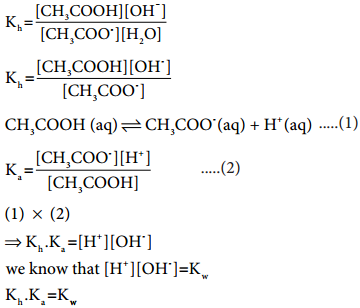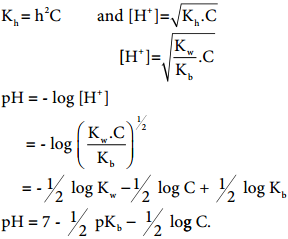Find free online Chemistry Topics covering a broad range of concepts from research institutes around the world.

## Salt Hydrolysis

When an acid reacts with a base, a salt and water are formed and the reaction is called neutralization. Salts completely dissociate in aqueous solutions to give their constituent ions. The ions so produced are hydrated in water. In certain cases, the cation, anion or both react with water and the reaction is called salt hydrolysis. Hence, salt hydrolysis is the reverse of neutralization reaction.

Salts of Strong Acid and a Strong Base

Let us consider the reaction between NaOH and nitric acid to give sodium nitrate and water.
NaOH(aq) + HNO3(aq) → NaNO3(aq) + H2O(l)

The salt NaNO3 completely dissociates in water to produce Na+ and NO3 ions.
NaNO3(aq) → Na+(aq) + NO3(aq)

Water dissociates to a small extent as
H2O(l) ⇄ H+(aq) + OH(aq)

Since [H+] = [OH], water is neutral
NO3 ion is the conjugate base of the strong acid HNO3 and hence it has no tendency to react

with H+. Similarly, Na+ is the conjugate acid of the strong base NaOH and it has no tendency to react with OH.

It means that there is no hydrolysis. In such cases [H+] = [OH] pH is maintained and, therefore, the solution is neutral.

Hydrolysis of Salt of Strong Base and Weak Acid (Anionic Hydrolysis)

Let us consider the reactions between sodium hydroxide and acetic acid to give sodium acetate and water.
NaOH (aq) + CH3COOH(aq) ⇄ CH3COONa(aq) + H2O(l)

In aqueous solution, CH3COONa is completely dissociated as below

CH3COONa (aq) → CH3COO(aq) + Na+(aq)

CH3COO is a conjugate base of the weak acid CH3COOH and it has a tendency to react with H+
from water to produce unionised acid.

There is no such tendency for Na+ to react with OH.

CH3COO(aq) + H2O(l) ⇄ CH3COOH(aq) + OH(aq) and therefore [OH]>[H+], in such cases, the solution is basic due to hydrolysis and the pH is greater than 7. Let us find a relation between the equilibrium constant for the hydrolysis reaction (hydrolysis constant) and the dissociation constant of the acid.Kh value in terms of degree of hydrolysis (h) and the concentration of salt (C) for the equilibrium can be obtained as in the case of ostwald’s dilution law. Kh = h2C and i.e [OH] = $$\sqrt{\mathrm{K}_{\mathrm{h}} \cdot \mathrm{C}}$$ and i.e [OH] = $$\sqrt{\mathrm{K}_{\mathrm{h}} \cdot \mathrm{C}}$$.

pH of salt solution in terms of Ka and the concentration of the electrolyte

pH + pOH = 14
pH = 14 – p OH = 14 – {- log [OH]}
= 14 + log[OH]Hydrolysis of Salt of Strong Acid and Weak Base (Cationic Hydrolysis)

Let us consider the reactions between a strong acid, HCl, and a weak base, NH4OH, to produce a salt, NH4Cl, and water.

HCl (aq) + NH4OH(aq) ⇄ NH4Cl(aq) + H2O(l)
NH4Cl(aq) → NH4+ + Cl(aq)

NH4+ is a strong conjugate acid of the weak base NH4OH and it has a tendency to react with with OH from water to produce unionised NH4OH shown below.

NH4+(aq) + H2O(l) ⇄ NH4OH(aq) + H+(aq)

There is no such tendency shown by Cl and therefore [H+]>[OH]; the solution is acidic and the pH
is less than 7.

As discussed in the salt hydrolysis of strong base and weak acid. In this case also, we can establish a relationship between the Kh and kb as Kh.Kb = Kw

Let us calculate the Kh value in terms of degree of hydrolysis (h) and the concentration of saltHydrolysis of Salt of Weak Acid and Weak Base (Anionic & Cationic Hydrolysis)

Let us consider the hydrolysis of ammonium acetate.
CH3COONH4(aq) → CH3COO(aq) + NH4+(aq)

In this case, both the cation (NH4+) and anion (CH3COO) have the tendency to
react with water

CH3COO + H2O ⇄ CH3COOH + OH
NH4+ + H2O ⇄ NH4OH + H+

The nature of the solution depends on the strength of acid (or) base i.e, if Ka > Kb; then the solution is acidic and pH < 7, if Ka < Kb; then the solution is acidic and pH < 7, if Ka < Kb; then the solution is basic and pH > 7, if Ka = Kb; then the solution is basic and pH > 7, if Ka = Kb; then the solution is neutral.

The relation between the dissociation constant (Ka, Kb) and the hydrolysis constant is given by the following
expression.

Ka.Kb.Kh = Kw

pH of the Solution

pH of the solution can be calculated using the following expression,
pH = 7 + 1/2 pKa – 1/2 pKb.